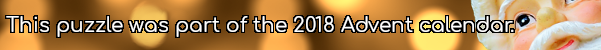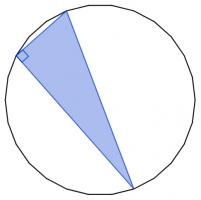mscroggs.co.uk
mscroggs.co.uksubscribe

# Puzzles

## 12 DecemberThere are 2600 different ways to pick three vertices of a regular 26-sided shape. Sometime the three vertices you pick form a right angled triangle.These three vertices form a right angled triangle.
Today's number is the number of different ways to pick three vertices of a regular 26-sided shape so that the three vertices make a right angled triangle.

## Dodexagon

In the diagram, B, A, C, D, E, F, G, H, I, J, K and L are the vertices of a regular dodecagon and B, A, M, N, O and P are the vertices of a regular hexagon.
Show that A, M and E lie on a straight line.

## Archive

Show me a random puzzle
▼ show ▼